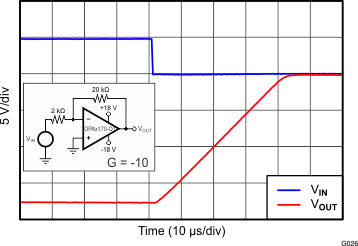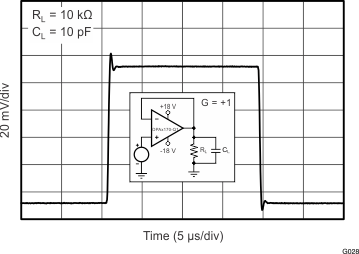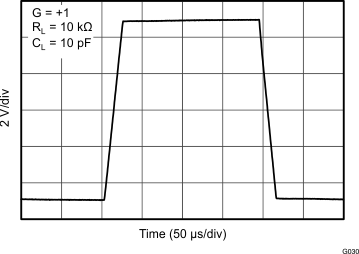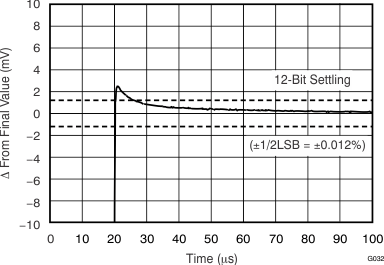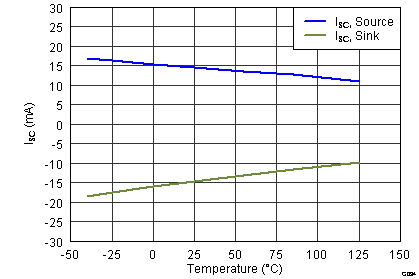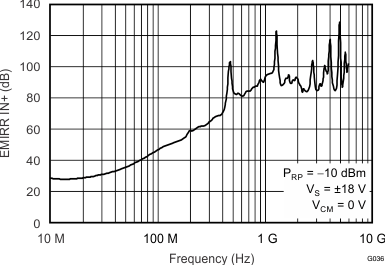SBOS834B December   2016  – November 2017

PRODUCTION DATA.

1. Features
2. Applications
3. Description
4. Revision History
5. Pin Configuration and Functions
6. Specifications
7. Detailed Description
1. 7.1 Overview
2. 7.2 Functional Block Diagram
3. 7.3 Feature Description
4. 7.4 Device Functional Modes
8. Application and Implementation
1. 8.1 Application Information
2. 8.2 Typical Application
9. Power Supply Recommendations
10. 10Layout
11. 11Device and Documentation Support
1. 11.1 Device Support
1. 11.1.1 Development Support
2. 11.2 Documentation Support
4. 11.4 Community Resources
6. 11.6 Electrostatic Discharge Caution
7. 11.7 Glossary
12. 12Mechanical, Packaging, and Orderable Information

• DGK|8
• DGK|8

6 Specifications

6.1 Absolute Maximum Ratings

over operating free-air temperature range (unless otherwise noted)(1)
MIN MAX UNIT
Supply voltage –20 20 V
Single supply voltage 40 V
Signal input pin voltage (V–) – 0.5 (V+) + 0.5 V
Signal input pin current –10 10 mA
Output short-circuit current(2) Continuous
Operating ambient temperature, TA –55 150 °C
Junction temperature, TJ 150 °C
Storage temperature, Tstg –65 150 °C
Stresses beyond those listed under Absolute Maximum Ratings may cause permanent damage to the device. These are stress ratings only, which do not imply functional operation of the device at these or any other conditions beyond those indicated under Recommended Operating Conditions. Exposure to absolute-maximum-rated conditions for extended periods may affect device reliability.
Short-circuit to ground, one amplifier per package.

6.2 ESD Ratings

VALUE UNIT
V(ESD) Electrostatic discharge Human-body model (HBM), per AEC Q100-002(1) ±4000 V
Charged-device model (CDM), per AEC Q100-011 ±750
AEC Q100-002 indicates that HBM stressing shall be in accordance with the ANSI/ESDA/JEDEC JS-001 specification.

6.3 Recommended Operating Conditions

over operating free-air temperature range (unless otherwise noted)
MIN MAX UNIT
VS Supply voltage (V+ – V–) 2.7 36 V
TA Operating temperature –40 125 °C

6.4 Thermal Information: OPA170-Q1

THERMAL METRIC(1) OPA170-Q1 UNIT
DBV (SOT-23)
5 PINS
RθJA Junction-to-ambient thermal resistance 245.8 °C/W
RθJC(top) Junction-to-case (top) thermal resistance 133.9 °C/W
RθJB Junction-to-board thermal resistance 83.6 °C/W
ψJT Junction-to-top characterization parameter 18.2 °C/W
ψJB Junction-to-board characterization parameter 83.1 °C/W
RθJC(bot) Junction-to-case (bottom) thermal resistance °C/W

6.5 Thermal Information: OPA2170-Q1

THERMAL METRIC(1) OPA2170-Q1 UNIT
DGK (VSSOP)
8 PINS
RθJA Junction-to-ambient thermal resistance 180 °C/W
RθJC(top) Junction-to-case (top) thermal resistance 55 °C/W
RθJB Junction-to-board thermal resistance 130 °C/W
ψJT Junction-to-top characterization parameter 5.3 °C/W
ψJB Junction-to-board characterization parameter 120 °C/W
RθJC(bot) Junction-to-case (bottom) thermal resistance °C/W

6.6 Thermal Information: OPA4170-Q1

THERMAL METRIC(1) OPA4170-Q1 UNIT
PW (TSSOP)
14 PINS
RθJA Junction-to-ambient thermal resistance 106.9 °C/W
RθJC(top) Junction-to-case (top) thermal resistance 24.4 °C/W
RθJB Junction-to-board thermal resistance 59.3 °C/W
ψJT Junction-to-top characterization parameter 0.6 °C/W
ψJB Junction-to-board characterization parameter 54.3 °C/W
RθJC(bot) Junction-to-case (bottom) thermal resistance °C/W

6.7 Electrical Characteristics

at TA = 25°C, VCM = VOUT = VS / 2, and RL = 10 kΩ connected to VS / 2 (unless otherwise noted)
PARAMETER TEST CONDITIONS MIN TYP MAX UNIT
OFFSET VOLTAGE
VOS Input offset voltage TA = 25°C 0.25 ±1.8 mV
TA = –40°C to 125°C ±2 mV
dVOS/dT Input offset voltage drift TA = –40°C to 125°C ±0.3 ±2 µV/°C
PSRR Input offset voltage vs power supply VS = 4 V to 36 V
TA = –40°C to 125°C
1 ±5 µV/V
Channel separation, dc 5 µV/V
INPUT BIAS CURRENT
IB Input bias current TA = 25°C ±8 ±15 pA
TA = –40°C to 125°C (OPA170-Q1 and OPA2170-Q1) ±3.5 nA
TA = –40°C to 125°C (OPA4170-Q1) ±16
IOS Input offset current TA = 25°C ±4 ±15 pA
TA = –40°C to 125°C (OPA170-Q1 and OPA2170-Q1) ±3.5 nA
TA = –40°C to 125°C (OPA4170-Q1) ±16
NOISE
Input voltage noise ƒ = 0.1 Hz to 10 Hz 2 µVPP
en Input voltage noise density ƒ = 100 Hz 22 nV/√Hz
ƒ = 1 kHz 19 nV/√Hz
INPUT VOLTAGE
VCM Common-mode voltage range(1) (V–) – 0.1 (V+) – 2 V
CMRR Common-mode rejection ratio VS = ±2 V, (V–) – 0.1 V < VCM < (V+) – 2 V
TA = –40°C to 125°C
90 104 dB
VS = ±18 V, (V–) – 0.1 V < VCM < (V+) – 2 V
TA = –40°C to 125°C
104 120 dB
INPUT IMPEDANCE
Differential 100 || 3 MΩ || pF
Common-mode 6 || 3 1012 Ω || pF
OPEN-LOOP GAIN
AOL Open-loop voltage gain VS = 4 V to 36 V
(V–) + 0.35 V < VO < (V+) – 0.35 V
TA = –40°C to 125°C
110 130 dB
FREQUENCY RESPONSE
GBP Gain bandwidth product 1.2 MHz
SR Slew rate G = 1 0.4 V/µs
tS Settling time To 0.1%, VS = ±18 V, G = 1 10-V step 20 µs
To 0.01% (12-bit), VS = ±18 V, G = 1
10-V step
28 µs
Overload recovery time VIN × Gain > VS 2 µs
THD+N Total harmonic distortion + noise G = 1, ƒ = 1 kHz, VO = 3 VRMS 0.0002%
OUTPUT
VO Voltage output swing from positive rail IL = 0 mA
VS = 4 V to 36 V
10 mV
IL sourcing 1 mA
VS = 4 V to 36 V
115 mV
VO Voltage output swing from negative rail IL = 0 mA
VS = 4 V to 36 V
8 mV
IL sinking 1 mA
VS = 4 V to 36 V
70 mV
VO Voltage output swing from rail VS = 5 V
RL = 10 kΩ
TA = –40°C to 125°C
(V–) + 0.03 (V+) – 0.05 V
RL = 10 kΩ
AOL ≥ 110 dB
TA = –40°C to 125°C
(V–) + 0.35 (V+) – 0.35 V
ISC Short-circuit current –20 17 mA
RO Open-loop output resistance ƒ = 1 MHz
IO = 0 A
900 Ω
POWER SUPPLY
VS Specified voltage range 2.7 36 V
IQ Quiescent current per amplifier IO = 0 A
TA = 25°C
110 145 µA
IO = 0 A
TA = –40°C to 125°C
155 µA
TEMPERATURE
Specified range –40 125 °C
Operating range –55 150 °C
The input range can be extended beyond (V+) – 2 V up to V+. For additional information, see Typical Characteristics and Application and Implementation.

Table 4. Characteristic Performance Measurements

DESCRIPTION FIGURE
Offset Voltage Production Distribution Figure 1
Offset Voltage Drift Distribution Figure 2
Offset Voltage vs Temperature Figure 3
Offset Voltage vs Common-Mode Voltage Figure 4
Offset Voltage vs Common-Mode Voltage (Upper Stage) Figure 5
Offset Voltage vs Power Supply Figure 6
IB and IOS vs Common-Mode Voltage Figure 7
Input Bias Current vs Temperature Figure 8
Output Voltage Swing vs Output Current (Maximum Supply) Figure 9
CMRR and PSRR vs Frequency (Referred-to-Input) Figure 10
CMRR vs Temperature Figure 11
PSRR vs Temperature Figure 12
0.1-Hz to 10-Hz Noise Figure 13
Input Voltage Noise Spectral Density vs Frequency Figure 14
THD+N Ratio vs Frequency Figure 15
THD+N vs Output Amplitude Figure 16
Quiescent Current vs Temperature Figure 17
Quiescent Current vs Supply Voltage Figure 18
Open-Loop Gain and Phase vs Frequency Figure 19
Closed-Loop Gain vs Frequency Figure 20
Open-Loop Gain vs Temperature Figure 21
Open-Loop Output Impedance vs Frequency Figure 22
Small-Signal Overshoot vs Capacitive Load (100-mV Output Step) Figure 23, Figure 24
No Phase Reversal Figure 25
Small-Signal Step Response (100 mV) Figure 28, Figure 29
Large-Signal Step Response Figure 30, Figure 31
Large-Signal Settling Time (10-V Positive Step) Figure 32
Large-Signal Settling Time (10-V Negative Step) Figure 33
Short-Circuit Current vs Temperature Figure 34
Maximum Output Voltage vs Frequency Figure 35
EMIRR IN+ vs Frequency Figure 36

6.9 Typical Characteristics

VS = ±18 V, VCM = VS / 2, RLOAD = 10 kΩ connected to VS / 2, and CL = 100 pF (unless otherwise noted)Figure 1. Offset Voltage Production Distribution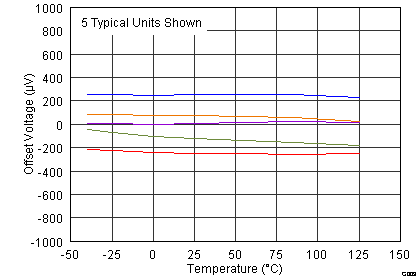Figure 3. Offset Voltage vs Temperature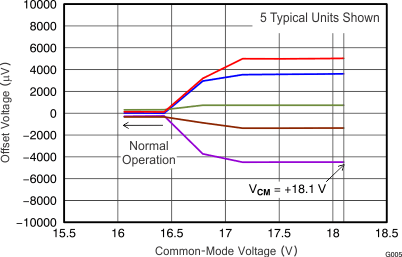Figure 5. Offset Voltage vs Common-Mode Voltage
(Upper Stage)Figure 7. IB and IOS vs Common-Mode Voltage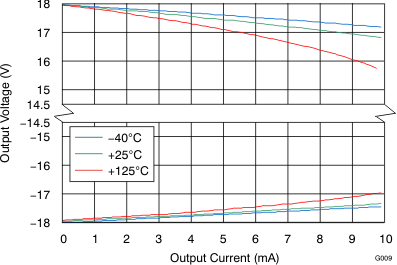Figure 9. Output Voltage Swing vs Output Current (Maximum Supply)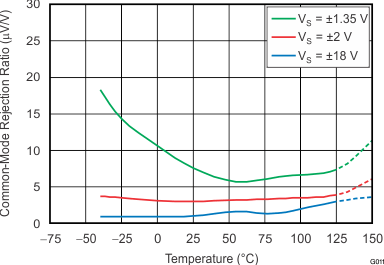Figure 11. CMRR vs Temperature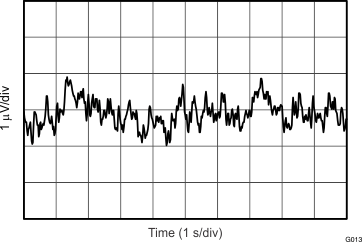Figure 13. 0.1-Hz to 10-Hz Noise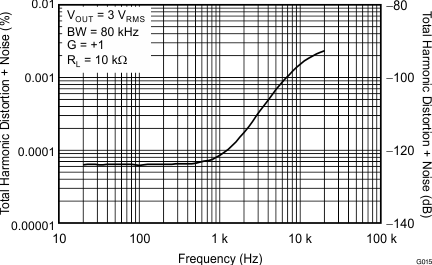Figure 15. THD + N Ratio vs Frequency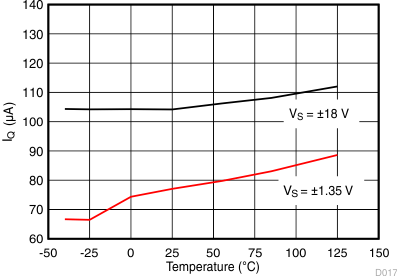Figure 17. Quiescent Current vs Temperature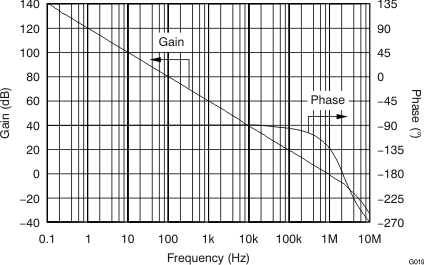Figure 19. Open-Loop Gain and Phase vs Frequency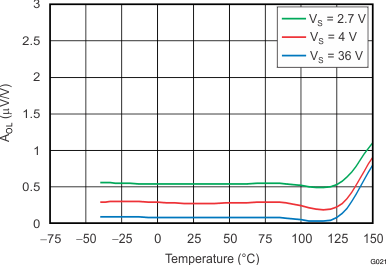Figure 21. Open-Loop Gain vs Temperature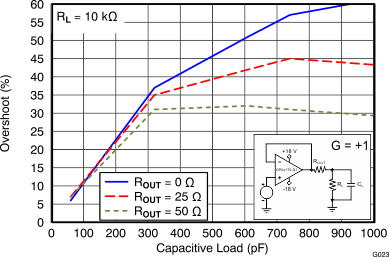100-mV output step
Figure 23. Small-Signal Overshoot vs Capacitive Load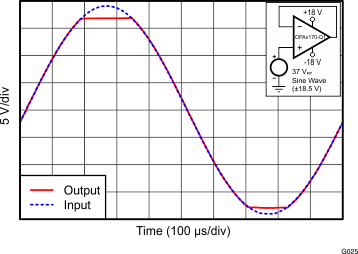Figure 25. No Phase Reversal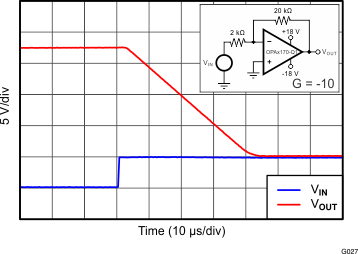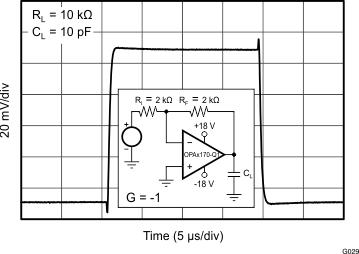Figure 29. Small-Signal Step Response (100-mV)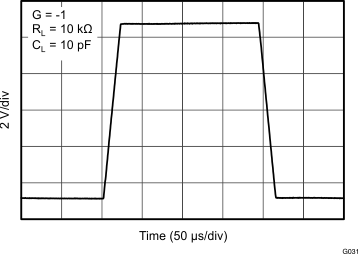Figure 31. Large-Signal Step Response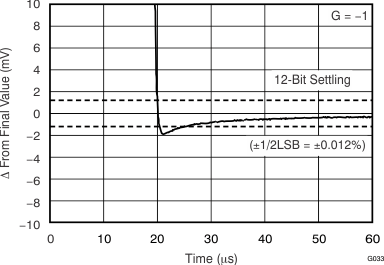10-V negative step
Figure 33. Large-Signal Settling Time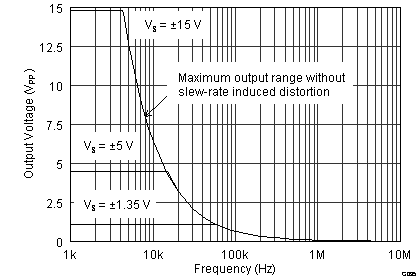Figure 35. Maximum Output Voltage vs Frequency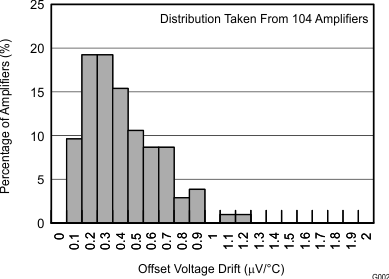Figure 2. Offset Voltage Drift Distribution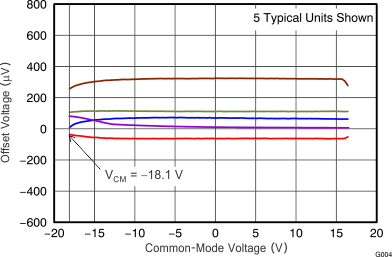Figure 4. Offset Voltage vs Common-Mode Voltage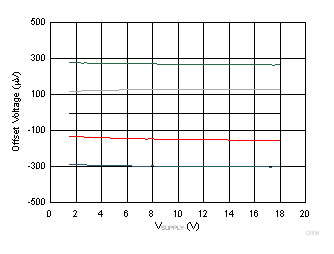Figure 6. Offset Voltage vs Power Supply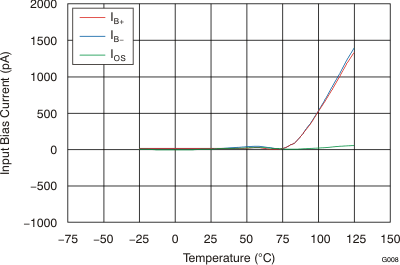Figure 8. Input Bias Current vs Temperature for Single and Dual Versions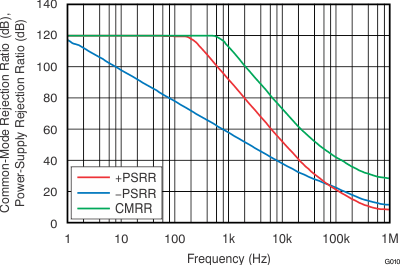Figure 10. CMRR and PSRR vs Frequency
(Referred to Input)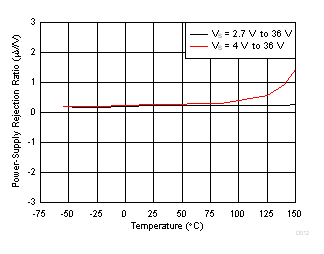Figure 12. PSRR vs TemperatureFigure 14. Input Voltage Noise Spectral Density vs FrequencyFigure 16. THD + N vs Output Amplitude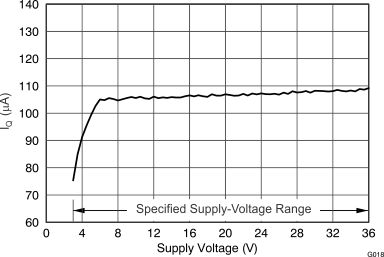Figure 18. Quiescent Current vs Supply Voltage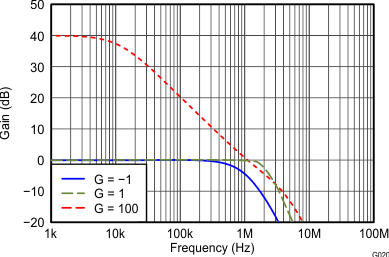Figure 20. Closed-Loop Gain vs Frequency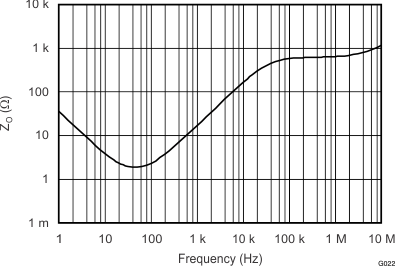Figure 22. Open-Loop Output Impedance vs Frequency100-mV output step
Figure 24. Small-Signal Overshoot vs Capacitive Load